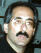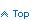# Programmatically Enumerate the values of an ENUM structure in VB.NET

It is common to use an Enum in VB.NET to enumerate a set of related values. For example, you can list the types of error messages your program logs using an enumeration such as:

```Public Enum ErrorLogMessageType
ExceptionMsg = 1
StackTrace = 2
ConnectionString = 3
SQLCommand = 4
OtherMessage = 5
End Enum
```

Then, you can reference the error message type using the more descriptive enumeration instead of using the unintuitive numeric value. Instead of referring to a message type using:

```   If ErrorMessageType = 1 Then
```

```   If ErrorMessageType = ErrorLogMessageType.ExceptionMsg Then
```

If you want to get the actual name of the enumeration value, ex, display the text ExceptionMsg instead of its value of 1, you could use the following:

```   ErrorLogMessageType.ExceptionMsg.ToString()
```

Sometimes you might need to loop through the Enumeration's values. You can do so using the GetValues method of the Enum class. GetValues takes a parameter which is the type of enumeration. To pass the type in, use VB's GetType function. The following code snippet illustrates how to loop through an enumeration.

```   Dim ErrorType As ErrorLogMessageType

For Each ErrorType In [Enum].GetValues(GetType(ErrorLogMessageType))
Dim strMsgType as String = ErrorType.ToString()

Select Case strMsgType
Case "SQLCommand"
...
Case "ConnectionString"
...
Case "ExceptionMsg"
...
End Select
Next
```

Now, what if you have an enumeration's value and you want to get the name of the enumeration constant? Suppose you have the value of "2" and you need to get the name.

```   Dim intValue as Integer = 2

[Enum].GetName(GetType(ErrorLogMessageType), intValue)
```

Will return "ConnectionString".